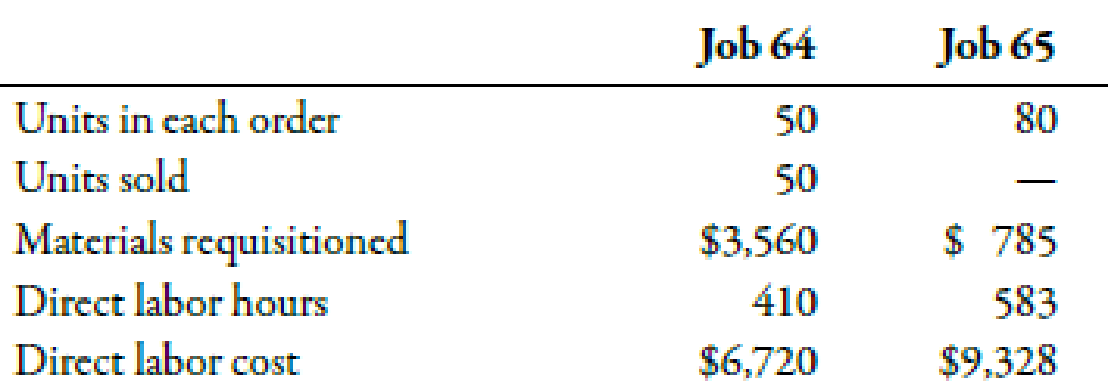Chapter 4, Problem 59P### Managerial Accounting: The Corners...

7th Edition
Maryanne M. Mowen + 2 others
ISBN: 9781337115773

#### Solutions

Chapter
Section### Managerial Accounting: The Corners...

7th Edition
Maryanne M. Mowen + 2 others
ISBN: 9781337115773
Textbook Problem
1 views

# (Appendix 4A) Unit Cost, Ending Work in Process, Journal EntriesDuring August, Leming Inc. worked on two jobs. Data relating to these two jobs follow:Overhead is assigned on the basis of direct labor hours at a rate of $11. During August, Job 64 was completed and transferred to Finished Goods. Job 65 was the only unfinished job at the end of the month.Required: 1. Calculate the per-unit cost of Job 64. 2. Compute the ending balance in the work-in-process account. 3. Prepare the journal entries reflecting the completion and sale on account of Job 64. The selling price is 175% of cost. (Note: Round all journal entry amounts to the nearest dollar.) 1. To determine Compute per unit cost of Job 64. Explanation Per Unit Cost: The cost incurred by the company to produce one unit of any product is known as per unit cost. It can be determined by dividing the total cost by the number of units produced. Use the following formula to calculate per unit cost: Per unit cost=Total costNumber of units Substitute$14,790 for total cost and 50 for the number of units in the above formula.

Per unit prime cost=$14,79050=$295.80

Therefore, per unit cost is \$295.80.

Working Note:

1

2.

To determine

Calculate ending balance in work in process.

3.

To determine

Pass the journal entries to record completion and sale on account of Job 64.

### Still sussing out bartleby?

Check out a sample textbook solution.

See a sample solution

#### The Solution to Your Study Problems

Bartleby provides explanations to thousands of textbook problems written by our experts, many with advanced degrees!

Get Started

#### Find more solutions based on key concepts